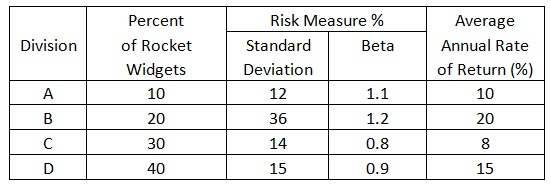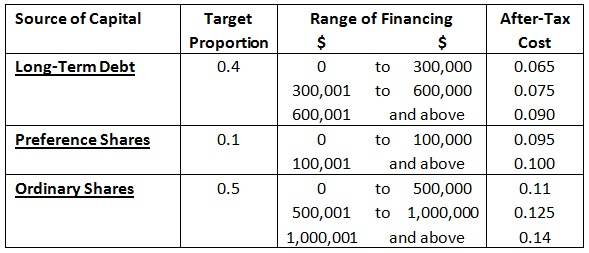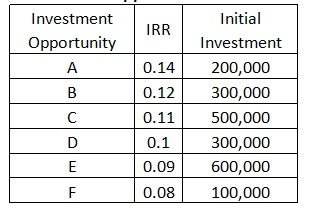Breaking points and ranges of total financing

PART A: (10 marks)

You have been asked to examine Grand Plomp Ltd, a maker of rocket widgets employed by NASA. The owners are wondering whether the return received is adequate to justify the risks taken in each division. You are to consider both divisional risk and return in your analysis and the given information has been collected from the past 15 years to use in your analysis.• Average annual market return: 12 per cent
• Average annual risk-free rate: 8 per cent

By using standard deviation and beta measures of risk, you are to rank projects in terms of their risk-adjusted return. Such divisions providing the lowest risk per unit of return would be preferred. The analysis can be conducted by using a 'total' definition of risk, or standard deviation, and an ’undiversifiable’ definition or risk or beta.

Given the beta, it is possible to evaluate the required rate of return. Moreover, given the proportion of each division in Global Gears, it is possible to compute the firm beta, return and risk-adjusted return. With this information, it is possible to find out whether Global Gears, as a whole, provides an adequate return to justify the risks taken by its investors.

You are required to:

• Place the relevant information.
• Choose the proper tool or equation.
• Organize and manipulate the data.
• Elucidate the solution.

PART B: (12.5 marks)

Misty Ltd wishes to find out its weighted marginal cost of capital. In preparing for this task, it has compiled the following data:a) Determine the breaking points and ranges of total financing associated with each source of capital;

b) By using the data developed determine the levels of total financing at which the firm's weighted average cost of capital (WACC) will change;

c) Compute the weighted average cost of capital and the weighted marginal cost (WMCC) for each range of total financing

d) By using the results all along with the information on the available investment opportunities shown below, compile the firm's investment opportunities schedule (IOS), plot this schedule and plot the weighted marginal cost of the capital schedule:Investment Opportunities Schedule

e) Which, if any of the available investments would you suggest that the firm accept? Describe your answer.

PART C: (7.5 marks)

Traditional project evaluation or capital budgeting analysis assumes a firm’s only choice is accept or refuse a program. In a real business situation, firms face many choices with respect to how to operate a project, both before it starts and after it is underway. Any time a firm has the capability to make choices, there is value added to the project in question – Traditional NPV analysis ignores this value. The study of real options tries to put a dollar value on the capability to make choices.

a) What are real options and how they are valued.
b) Discuss the given:

Locate the given article: IRREVERSIBILITY, UNCERTAINTY, AND INVESTMENT (Robert S Pindyck – Massachusetts Institute of Technology March – 1990 – old but gold)

Most of the major investment expenditures have two significant characteristics which together can dramatically affect the decision to invest. First, the expenditures are largely irreversible; the firm can’t disinvest, thus the expenditures must be viewed as sunk costs. Second, the investments can be delayed, giving the firm an opportunity to wait for new information regarding prices, costs and other market conditions before it commits resources.

c) Compute the given:

Pindyck supplies a simple two-period example to exemplify how irreversibility can affect an investment decision and how option pricing methods can be used to value a firm’s investment opportunity, and determine whether or not the firm should invest.

Using the given example replicate Pyndick’s two-period example.

Consider a firm's decision to irreversibly invest in a widget factory. The factory can be built instantly, at a cost of \$7m, and will generate 1000 widgets per year forever, with zero operating cost. Currently the price of widgets is \$700, but next year the price will change. With probability .6it will rise to \$800, and with probability (l-q) it will fall to \$600. The price will then remain at this new level forever. Assume that this risk is fully diversifiable, so that the firm can discount future cash flows using the risk-free rate, which we will take to be 10 percent.

Solution Preview :

Reference No:- TGS018

Now Priced at \$40 (50% Discount)

Recommended (96%)

Rated (4.8/5)